https://doi.org/10.5194/esd-13-1715-2022
https://doi.org/10.5194/esd-13-1715-2022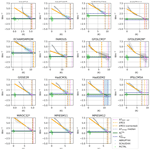# Potential for bias in effective climate sensitivity from state-dependent energetic imbalance

Benjamin M. Sanderson and Maria Rugenstein
Abstract

To estimate equilibrium climate sensitivity from a simulation where a step change in carbon dioxide concentrations is imposed, a common approach is to linearly extrapolate temperatures as a function of top-of-atmosphere energetic imbalance to estimate the equilibrium state (“effective climate sensitivity”). In this study, we find that this estimate may be biased in some models due to state-dependent energetic leaks. Using an ensemble of multi-millennial simulations of climate model response to a constant forcing, we estimate equilibrium climate sensitivity through Bayesian calibration of simple climate models which allow for responses from subdecadal to multi-millennial timescales. Results suggest potential biases in effective climate sensitivity in the case of particular models where radiative tendencies imply energetic imbalances which differ between pre-industrial and quadrupled CO2 states, whereas for other models even multi-thousand-year experiments are insufficient to predict the equilibrium state. These biases draw into question the utility of effective climate sensitivity as a metric of warming response to greenhouse gases and underline the requirement for operational climate sensitivity experiments on millennial timescales to better understand committed warming following a stabilization of greenhouse gases.

Share
Dates
1 Introduction

Equilibrium climate sensitivity (ECS) is the theoretical equilibrium increase in global mean temperature experienced in response to an instantaneous doubling in Earth's carbon dioxide concentrations over pre-industrial levels. Introduced as a metric of response of the Earth System to greenhouse gases in the early years of computational climate science , it remains a very common metric of the sensitivity of the Earth to greenhouse gas forcing .

Measuring ECS in a coupled climate model, however, is difficult owing to the time required for the equilibration of the system to a change in forcing necessitating simulations of multiple millennia to obtain a near-equilibrated estimate of temperature response . The computational burden of conducting such simulations implies that standard practice for model assessment is to measure an “effective climate sensitivity” (EffCS) using feedbacks extrapolated from those simulated in the first 150 years forced with a step-wise quadrupling of CO2 .

A core assumption in the calculation of EffCS is that the system will ultimately stabilize in a state of energetic balance . However, in practice a number of models exhibit energetic radiative top-of-atmosphere imbalances in the control state in both CMIP5 and CMIP6 , and as such the effective climate sensitivity is calculated using net flux anomalies relative to the control mean top-of-atmosphere net radiative fluxes. However, it remains untested as to whether such models will ultimately converge to the same state of imbalance.

In the present study, we consider an alternative approach for calculating climate sensitivity from a climate simulation in which there is a step change in carbon dioxide concentrations. We consider how the method of calculating effective climate sensitivity, either from initial response or from millennial-scale simulations, may be potentially subject to biases arising from assumptions regarding the equilibrated radiative state. Finally, we consider how these uncertainties relate to our confidence in the relationship between transient and equilibrium climate feedbacks.

We consider the role of non-equilibrated models in the context of recent research, which has highlighted potential uncertainties in the EffCS approximation of ECS – studies have found that net radiative feedbacks can exhibit both timescale and state dependencies (e.g., ) both of which draw into question the implicit constant feedback assumption used to calculate EffCS.

The LongRunMIP project set out in part to quantify this error by running a subset of Earth system models (ESMs) in idealized carbon dioxide perturbation experiments with simulations of millennial-timescale response . Initial studies compared the EffCS as derived using the first 150 years of the simulation with that derived using the last 15 % of warming in multi-thousand-year experiments, finding that the accuracy of the EffCS varied by model, but the two methods differed by 5 %–37 % in the estimate of ECS . A follow-up study considered a range of approaches for characterizing feedbacks on different timescales and found that feedbacks assessed in the period of 100–400 years after the initial quadrupling of CO2 concentrations may provide a practical prediction of equilibrium response accurate within 5 % or less. They found also, however, that there were large inconsistencies in some models between estimates of climate sensitivity derived from extrapolation to radiative equilibrium and those methods which relied on a fitting of exponentially decaying temperature trend, leaving uncertainty in the best practice for integrating model-derived EffCS distributions into uncertainty in long-term warming trajectories.

A general assessment of the likely range of EffCS (which itself informed the , assessed likely EffCS range) rested strongly on combined historical and paleo-evidence, contributing to the headline result that values of EffCS of greater than 4.7 K are unlikely. These findings somewhat challenge the use of the CMIP6 ensemble of climate models as a proxy for climate projection uncertainty in assessment, given approximately one-third of the ensemble have apparent EffCS values of greater than 4.7 K – leading to arguments that such “hot models” should be excluded from assessment .

So can these models be ruled out? Although studies suggest that post-1980 warming may help constrain the transient climate response , recent historical warming alone is only weakly correlated with EffCS in the CMIP5 and CMIP6 ensemble . In the present study, we find that this might in part be due to the fact that a key assumption in EffCS (that the model will return to the radiative balance observed in the control simulation) may not hold in a number of CMIP class models.

2 Methods

We consider fits of a simple multi-timescale model to idealized climate change experiments from LongRunMIP , which in general provide an estimate of the multi-millennial response of the Earth System to a constant radiative forcing level. The Supplement also illustrates results from CMIP5 and CMIP6 , but in general these simulations are insufficiently long to constrain the simple model response.

We assume that the temperature and radiative response to a step change in forcing can be modeled by a sum of exponential decay terms, a basis set which is consistent with the general solution of two-layer simple climate models and one which holds for the solution of a number of proposed multi-layer linear energy balance models in response to constant forcings . It has been shown also that some non-linear models have a solution set which can also be expressed in the same exponential basis . We consider N exponential response modes, such that

$\begin{array}{}\text{(1a)}& {T}_{p}\left(t\right)& =\sum _{n=\mathrm{1}}^{N}{S}_{n}\left(\mathrm{1}-{e}^{-\left(t/{\mathit{\tau }}_{n}\right)}\right)+{T}_{\mathrm{0}},\text{(1b)}& {R}_{p}\left(t\right)& =\sum _{n=\mathrm{1}}^{N}{R}_{n}\left(-{e}^{-\left(t/{\mathit{\tau }}_{n}\right)}\right)+{R}_{\mathrm{extrap}}^{\mathrm{4}x},\end{array}$

where Tp(t) and Rp(t) are the global annual mean surface temperature and net top-of-atmosphere radiative flux time series in response to an assumed F4x=7.2 W m−2 step change in forcing (F4x corresponding approximately to a quadrupling of CO2; ), τn is the decay time associated with the timescale n, Sn and Rn are scaling factors, and T0 and ${R}_{\mathrm{extrap}}^{\mathrm{4}x}$ are constant terms. T0 represents the pre-pulse temperature, taken here as the mean temperature in the last available 500 years of the control simulation. ${R}_{\mathrm{extrap}}^{\mathrm{4}x}$ is the radiative flux imbalance as t→∞ in the forced simulation and is calibrated during the calculation.

We distinguish between the radiative flux imbalance, ${R}^{{\mathrm{CTRL}}_{\mathrm{0}}}$, in the Pre-Industrial Control Simulation (PICTRL) and imbalance ${R}_{\mathrm{extrap}}^{\mathrm{4}x}$ in the asymptotic limit of the instantaneous CO2 quadrupling experiment (ABRUPT4X). For models which provided constant forcing extensions of transient experiments, we assume ${R}_{\mathrm{extrap}}^{\mathrm{4}x}$ is a fixed property of the fitted pulse-response function. ${R}^{{\mathrm{CTRL}}_{\mathrm{0}}}$ is calculated as the time average of net top-of-atmosphere (TOA) flux from the last 500 years of PICTRL. In fully equilibrated models with no energetic leaks, it would be expected that ${R}_{\mathrm{0}}^{\mathrm{CTRL}}=\mathrm{0}$, but it has been noted previously that this is not always the case and small energetic imbalances remain in some models even after the model global mean temperature trends have ceased .

Existing studies differ in the number of independent equilibration timescales (N) which describe the joint evolution of top-of-atmosphere net radiative balance (Rp(t)) and the global mean surface temperature (Tp(t)) in response to a step change in forcing, generally using two or three timescales . Here we consider solutions ranging from two to five timescales allowing for a range of thermal responses corresponding approximately to subdecadal, decadal, centennial, millennial and multi-millennial (see Tables 1 and 2).

Table 1Table showing the included modes from Table 2 for each model variant considered.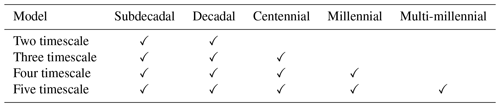Table 2Parameters and prior ranges considered in the Bayesian calibration of Eq. (1a). Parameters marked * are optionally included according to the model under consideration (see Table 1).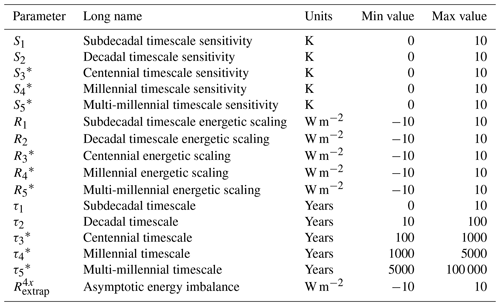For LongRunMIP models which provide an experiment with an abrupt quadrupling of CO2 (ABRUPT4X hereafter), we take Tp(t) and Rp(t) as global annual mean values from ABRUPT4X simulations to directly calibrate the parameters in Eqs. (1) and (1b). Some models, however, do not provide ABRUPT4X, instead providing constant forcing extensions of other climate change experiments (see ). For these models, we further assume a linear pulse-response formulation to represent the thermal global mean response to the corresponding forcing time series as the convolution of the thermal response to a step change in forcing, combined with the forcing time series itself .

$\begin{array}{}\text{(2a)}& T\left(t\right)& =\sum _{{t}^{\prime }=\mathrm{1}}^{t}{T}_{p}\left(t-{t}^{\prime }\right)\frac{F\left({t}^{\prime }\right)-F\left({t}^{\prime }-\mathrm{1}\right)}{{F}_{\mathrm{4}x}},\text{(2b)}& R\left(t\right)& =\sum _{{t}^{\prime }=\mathrm{1}}^{t}{R}_{p}\left(t-{t}^{\prime }\right)\frac{F\left({t}^{\prime }\right)-F\left({t}^{\prime }-\mathrm{1}\right)}{{F}_{\mathrm{4}x}},\end{array}$

where F(t) is the forcing time series of the corresponding experiment. Here we assume approximate logarithmic forcing dependencies for carbon dioxide (a dependency which is an empirical outcome of more complex radiative transfer models; ) and integrated forcing estimates for the one model (ECEARTH) which extended a multi-forcer future scenario experiment in LongRunMIP. The latter forcing estimate is an approximation with central estimates for aerosol and greenhouse gas forcing rather than model-specific values, but the effective forcing time series experienced by ECEARTH under RCP85 is not knowable without dedicated simulations .

## 2.1 Bayesian calibration of model response parameters

We fit the response equations detailed in Eqs. (2a) and (2b) to the output of each ensemble member's global mean radiative flux and surface temperature time series using a Markov chain Monte Carlo (MCMC) optimizer (; as implemented in the “lmfit” Python module), sampling models which allow for a range of $N=\left[\mathrm{2},\mathrm{3},\mathrm{4},\mathrm{5}\right]$ representative decay timescales.

3 Results

## 3.1 Assessment of model response timescale

The following section is used to assess the simplest acceptable multi-timescale model for the emulation of different ESMs in the LongRunMIP archive. We quantify this using the root mean square error (RMSE) associated with the least-square fit optimization (assessed as the best performing member of the MCMC posterior solution). If the addition of an additional, longer timescale in the fit corresponds to a reduction in combined RMSE of 0.5 % or more, the longer timescale model is used.

The performance of fitted multi-timescale models for GMT (global annual mean surface temperature) and NET (global annual mean net top-of-atmosphere radiative imbalance) time series is summarized in Fig. 1, which shows the combined error in the fits for GMT and NET associated with the absolute least-square fit for each of the model variants described in Table 1. The associated time series for the best fitted model in the context of the original model data for GMT and NET are shown in the Appendix (Figs. A1 and A2).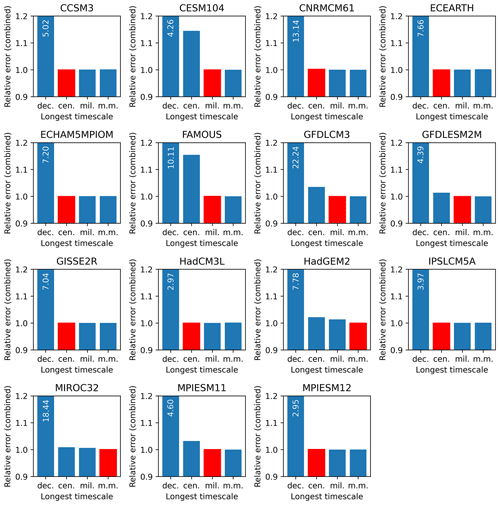Figure 1Illustration of the root mean square error for the fit to global mean temperature and net TOA radiative balance using models allowing for a range of timescales. Dec., Cen., Mil. and m.m. are decadal-, centennial-, millennial- and multi-millennial-timescale models, respectively. RMSE values for each variable (NET and GMT) are normalized relative to the best overall fit for that variable, each multiplied by 0.5 to give a combined error. The shortest timescale model with errors within 0.5 % tolerance of the overall best performing model is illustrated in red. Included modes and parameter priors are detailed in Tables 1 and 2. In cases where the error is truncated by the vertical axis, the value is printed in white.

We find that for all LongRunMIP models, the N=2 timescale model performs significantly worse than N≥3 timescale models allowing for centennial and longer response timescales. This is both evident by the significantly larger best fit errors (Fig. 1) as well as visibly poor fits (Figs. A1 and A2).

Differences between the $N=\mathrm{3},\mathrm{4}$ and 5 timescale models are dependent on the ESM being fitted. For some models (CCSM3, CNRMCM61, ECEARTH, ECHAM5MPIOM, GISSE2R, HadCM3L, IPSLCM5A, MPIESM12), no significant improvement in fit is seen beyond the centennial timescale model (Fig. 1). For other models, fits are further improved by allowing a millennial (CESM104, FAMOUS, GFDLCM3) or multi-millennial timescale (HadGEM2, MIROC32). Parameters associated with the best-fitting models are listed in Table A1, and fitted MCMC ensembles corresponding to the selected class of model illustrated in red in Fig. 1 are carried through for the remainder of the study.

## 3.2 Assessment of climate sensitivity

The conventional effective climate sensitivity (EffCS) is calculated using the first 150 years of simulation, linearly extrapolating GMT as a function of NET to ${R}_{\mathrm{0}}^{\mathrm{CTRL}}$. Control global mean temperatures and TOA energetic imbalances are expressed as anomalies relative to T0. We assess errors EffCS due to state-dependent radiative imbalance by calculating EffCScorr, where feedbacks in the first 150 years are instead linearly extrapolated to ${R}_{\mathrm{4}x}^{\mathrm{extrap}}$.

A third estimate of equilibrium warming, ΔTbest−est, follows , by calculating the effective climate sensitivity based on the years corresponding to the last 15 % of warming in the simulation (that is, for all years following the point when the simulation first exceeds 85 % of the average global mean temperature anomaly in the last 20 years of the ABRUPT4X simulation). For models which do not directly provide ABRUPT4X (GFDLCM3, GFDLESM2M and MIROC32), ΔTbest−est is calculated by scaling by the ratio of radiative forcing in ABRUPT4X relative to that in the multi-thousand-year constant forcing period in the experiment provided (following ; see Table 3).

Table 3Table showing assumed forcing evolution for experiments in LongRunMIP. * Logarithmic CO2 forcing dependency is assumed following . ${}^{**}$ Fhistorical(t) and FRCP85(t) forcing are taken according to .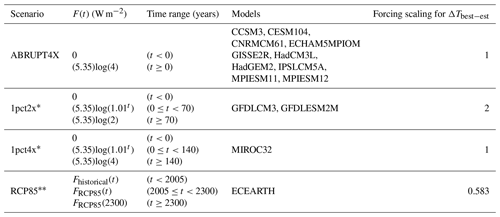We finally calculate a fourth estimate of climate sensitivity ΔTextrap as in Eq. (3) in the equilibrated (ABRUPT4X) simulation using the ensemble of fitted parameters from Bayesian calibration of Eq. (1), using again global mean temperature anomalies from ABRUPT4X relative to T0 (taken as mean temperatures over the last 100 years of PICTRL).

$\begin{array}{}\text{(3)}& {T}_{\mathrm{extrap}}=\sum _{n=\mathrm{1}}^{N}{S}_{n}+{T}_{\mathrm{0}}\end{array}$

We estimate the long-term radiative imbalance in the ABRUPT4X simulation from the fitted values for ${R}_{\mathrm{extrap}}^{\mathrm{4}x}$ (along with Rn, the amplitude of the decay in forcing at the timescale corresponding to τn) from Eq. (1b). Previous studies have assumed in the calculation of ΔTbest−est that ${R}_{\mathrm{extrap}}^{\mathrm{4}x}={R}_{\mathrm{0}}^{\mathrm{CTRL}}$ , an assumption we test here.

We follow convention by reporting climate sensitivities for a doubling of carbon dioxide from pre-industrial levels. As such, we follow standard practice in dividing ABRUPT4X sensitivities by 2 to obtain EffCS, ΔTextrap and ΔTbest−est , though we note that in some models this approximation introduces minor errors (; these are not the focus of the present study).

## 3.3 Relevance of energetic leakages

We consider first the radiative tendencies of the models in the climate change experiments, compared with the control state. Figure 2 shows the evolution of the top-of-atmosphere net radiative imbalance in the LongRunMIP climate change experiments, as well as the control simulation – together with the projected evolution of a simulated ABRUPT4X simulation using the fitted multi-timescale model. We note that there is significant model diversity in the behavior of models in the approach to equilibrium. Some models (CESM104, GISSE2R, GFDLESM2M, GFDLCM3 and MPIESM11) behave as expected, showing ${R}_{\mathrm{0}}^{\mathrm{CTRL}}=\mathrm{0}$ and ${R}_{\mathrm{4}x}^{\mathrm{extrap}}=\mathrm{0}$ (Fig. 2).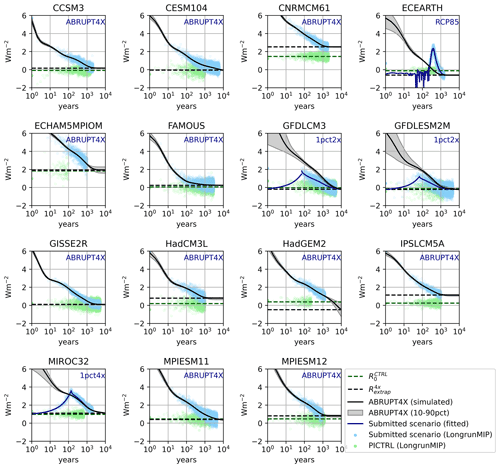Figure 2Top-of-atmosphere net radiative imbalance plotted as a function of time (log scale) for the members of the LongRunMIP ensemble. Dashed green line shows the control radiative imbalance $\left({R}_{\mathrm{0}}^{\mathrm{CTRL}}\right)$, while dashed black line shows the predicted ABRUPT4X radiative imbalance $\left({R}_{\mathrm{extrap}}^{\mathrm{4}x}\right)$. Semi-transparent blue and green points show annual mean upgoing net radiative flux from PICTRL and the submitted simulation (printed in blue text), respectively. Black line shows the simulated response to ABRUPT4X for the multi-timescale model, while shaded gray regions and thin lines show the 10th and 90th percentiles of the fitted ensemble projections for ABRUPT4X. If the submitted simulation was not ABRUPT4X, the thick blue line shows the MCMC posterior median TOA time series for the submitted simulation using the chosen multi-timescale model (see Table 1).

A second class of model exhibits a radiative imbalance in the control simulation, but the ABRUPT4X simulation converges to the same state (${R}_{\mathrm{0}}^{\mathrm{CTRL}}={R}_{\mathrm{4}x}^{\mathrm{extrap}}\ne \mathrm{0}$ e.g., MIROC32, MPIESM11). Finally, a third class appears to converge to different states in PICTRL and ABRUPT4X (${R}_{\mathrm{0}}^{\mathrm{CTRL}}\ne {R}_{\mathrm{4}x}^{\mathrm{extrap}}$, e.g., CCSM3, CNRMCM61, ECEARTH, HadCM3L, MIROC32, MPIESM12 and IPSLCM5A) – implying that effective climate sensitivity may be biased in these models if calculated assuming that the ABRUPT4X simulation is tending towards the equilibrium radiative state of the PICTRL simulation.

Figures 3 and 4 show the impact of these biases on the derived value for equilibrium climate sensitivity. The relationship between temperature and TOA fluxes for the fitted multi-timescale models for ABRUPT4X simulations in the LongRunMIP archive is presented in Fig. 3, while Fig. 4 shows the temperature evolution as a function of time.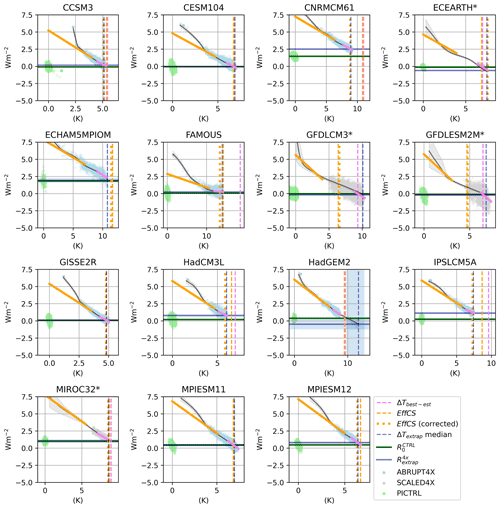Figure 3Global mean net radiative imbalance as a function of surface temperature for different members of the LongRunMIP archive. Vertical axis shows absolute top-of-atmosphere net radiative imbalance; horizontal axis shows surface temperature relative to the final 500 years of the control simulation. Models marked “*” did not provide ABRUPT4X directly (see Table 3). Solid black lines show the median simulation of ABRUPT4X for the fitted MCMC posterior of the multi-timescale model; shaded gray areas show 5 %–95 % confidence intervals. Light blue points are individual years from ABRUPT4X (if available). For * models, gray points show years in the latter portion of the simulation after which forcing is constant, scaled according to Table 3. Light green points are annual means from PICTRL. Yellow solid line shows the regression fit in years 0–150 for the original ABRUPT4X data if available (or simulated ABRUPT4X median model for models marked “*”), corresponding to the EffCS dashed yellow vertical line and EffCS (corrected) dotted yellow vertical line. Purple solid line shows regression fit to the last 15 % of warming following , corresponding to the ΔTbest−est vertical dashed line. Horizontal green line shows PICTRL net energy imbalance averaged over the final 500 years of the simulation. Horizontal solid blue line shows ${R}_{\mathrm{extrap}}^{\mathrm{4}x}$, while vertical dashed blue line shows ΔTextrap; shaded areas illustrate uncertainty in these values.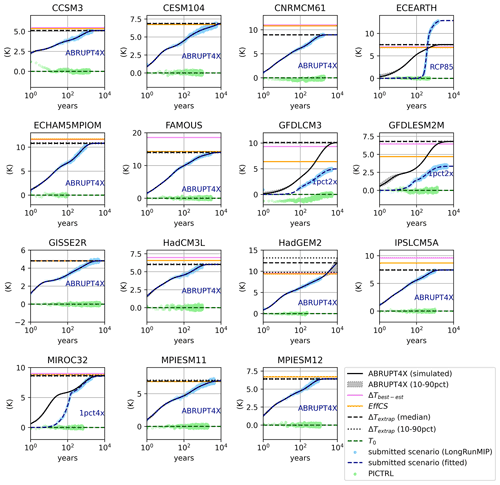Figure 4Global mean temperature anomaly with respect to the last 500 available years of the PICTRL simulation, plotted as a function of time (log scale) for the members of the LongRunMIP ensemble. Green points show annual global mean surface temperature anomalies from the LongRunMIP PICTRL simulation, while blue points show data from the submitted climate change experiment (printed in blue text for each model). Thick blue lines show the median top-of-atmosphere time series using the MCMC posterior fit for the multi-timescale model selected to represent the corresponding ESM (see Sect. 2 and Fig. 1). Black lines show the median response of the fitted multi-timescale model to an ABRUPT4X forcing, while shaded gray regions and thin dotted lines show the 10th and 90th percentiles of the fitted ABRUPT4X ensemble projections. Dashed black horizontal line illustrates ΔTextrap (median), yellow solid line is EffCS, pink solid is ΔTbest−est and dashed green line shows T0. Readers should note y axis differs by subplot.

Models with exact agreement between ${R}_{\mathrm{0}}^{\mathrm{CTRL}}$ and ${R}_{\mathrm{4}x}^{\mathrm{extrap}}$ also tend to exhibit similar values for ΔTbest−est and ΔTextrap, and in cases where there is little or no difference in feedbacks in the early and late stages of the simulation (e.g., CESM104, GISSE2R, MPIESM11), EffCS is also similar to ΔTbest−est and ΔTextrap. Other models (e.g., ECEARTH, ECHAM5MPIOM, FAMOUS, GFDLCM3, GFDLESM2M, IPSLCM5A) show significant differences in early and late stage feedbacks, manifested as a ΔTbest−est, which differs from EffCS.

Models with significant differences between ${R}_{\mathrm{0}}^{\mathrm{CTRL}}$ and ${R}_{\mathrm{4}x}^{\mathrm{extrap}}$ (CNRMCM61, FAMOUS, ECEARTH, HadCM3L, IPSLCM5A, MPIESM12) exhibit similar biases in both ΔTbest−est and EffCS. For example, CNRMCM61 exhibits relatively constant feedbacks on century and millennial timescales, so ΔTbest−est and EffCS are similar (5.42 and 5.51 K, respectively), but ΔTextrap, which is well fitted by the data, is significantly lower (4.47±0.01 K; Fig. 4 and Table 4) due to the differing estimated equilibrium energetic imbalance in ABRUPT4X and PICTRL simulations. The fitting process for HadGEM2 determined that a multi-millennial response mode was necessary, which remains unconstrained by the fit, so it is not possible to estimate ΔTextrap with confidence for this model (the simulation length for HadGEM2 is 1299 years, so it remains possible that a 5000-year simulation as provided by a number of other models could rule out the need for the multi-millennial response mode).

Table 4Fitted parameters and uncertainties for the LongRunMIP experiments. Median values, with 5th and 95th percentiles in brackets where relevant. The “Difference” column shows EffCScorrEffCS. EffCScorr is not calculated for HadGEM2 due to large uncertainties in ${R}_{\mathrm{extrap}}^{\mathrm{4}x}$.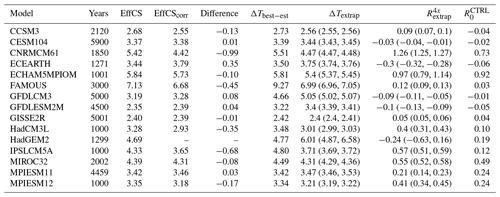Of these models with apparently state-dependent energetic balance, some (HadCM3L, FAMOUS, ECEARTH) appear to show a control simulation where ${R}_{\mathrm{0}}^{\mathrm{CTRL}}\approx \mathrm{0}$ but an ABRUPT4X simulation which converges to a state of energetic imbalance (Fig. 2). This, in turn introduces a source of potential bias in the estimate of effective climate sensitivity if the system is converging to a non-equilibrated state, implying that the control simulation may be tuned to exhibit energetic balance but the equilibrated 4xCO2 state is subject to an energy leak. A particularly extreme example is FAMOUS, where a small difference in extrapolated energetic balance, combined with a large feedback parameter, results in a much larger values of ΔTbest−est (9.27 K) than ΔTextrap (6.99 K; see Table 4 and Fig. 4) or EffCS (7.13 K).1 Similarly for HadCM3L, the fitted extrapolated sensitivity ΔTextrap (3.03 K; see Table 4 and Fig. 4) is lower than ΔTbest−est (3.49 K) and EffCS (3.29 K).

ΔTextrap differs from EffCS both due to the presence of state-dependent energetic biases but also due to feedbacks which occur over the multi-thousand-year timescales resolved in the LongRunMIP experiments. We can isolate the bias in EffCS induced by state-dependent energetic imbalance in the LongRunMIP cases by using a different extrapolated energetic state (Figs. 5 and 3). As in the standard calculation of EffCS, we take a least-squares linear fit of temperature as a function of N in the first 150 years but instead linearly extrapolate to $N={R}_{\mathrm{extrap}}^{\mathrm{4}x}$ rather than $N={R}_{\mathrm{0}}^{\mathrm{CTRL}}$ in the standard calculation to produce a bias-corrected EffCScorr. We find that two models in LongRunMIP are significantly impacted by this correction (see Figs. 5 and A3): CNRMCM61 (EffCS =5.42 K, EffCScorr=4.42 K) and IPSLCM5A (EffCS =4.33 K, EffCScorr=3.65 K). A number of other models are impacted to a lesser extent (see Table 4).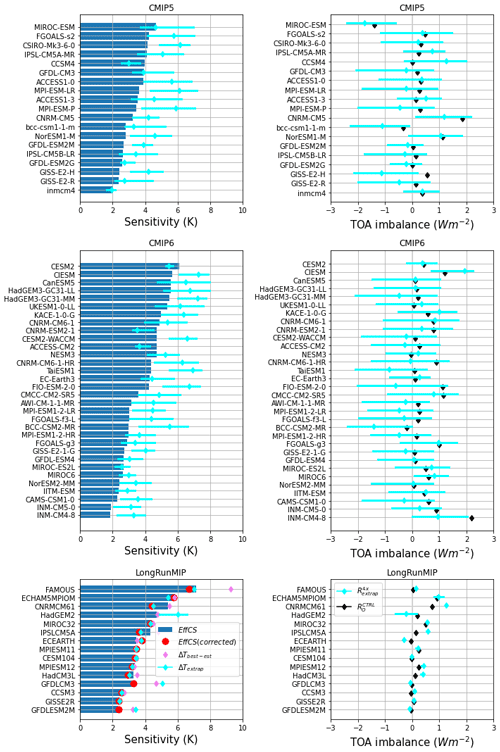Figure 5Bar plots summarizing results for three model ensembles, CMIP5 (top row), CMIP6 (middle row) and LongRunMIP (bottom row). Left-hand column shows different estimates of equilibrium climate sensitivity. Solid blue bars show EffCS (see text). Light blue diamond and whiskers show the median, 5th and 95th percentiles of ΔTextrap. For LongRunMIP, ΔTbest−est (following ), is shown in violet diamonds, while EffCScorr is shown with red circles. The right-hand column shows ${R}_{\mathrm{extrap}}^{\mathrm{4}x}$ (light blue diamond and whiskers) and ${R}_{\mathrm{0}}^{\mathrm{CTRL}}$ (black diamonds).

The analysis was repeated for the wider CMIP5 and CMIP6 ensembles. However, the standard CMIP5 and CMIP6 simulations are insufficiently long to fit response timescales of centennial or longer; hence ΔTextrap (or ${R}_{\mathrm{extrap}}^{\mathrm{4}x}$) is not constrained using the multi-timescale fitting approach (see Fig. 5). It is notable that flux imbalances are present in the control state of a number of models in both CMIP5 and CMIP6, but longer simulations are required to assess whether these represent structural imbalances or an insufficiently long spinup. The centennial and longer timescales are not constrained in 150-year simulations; hence it is not possible to estimate ΔTextrap and ${R}_{\mathrm{extrap}}^{\mathrm{4}x}$ with any confidence. We note, however, that in most cases the uncertainties in the fitted three-timescale solution generally allow for equilibrium values which are higher than the effective climate sensitivity as assessed over the first 150 years of simulation. Only a small number of models allow for fitted solutions which have a lower ΔTextrap than the EffCS (CESM2, CCSM4, MIROC5, CNRMESM2.1, ACCESS-CM2). One of these cases (CNRMCM6.1) is a close relative of the CNRMESM2.1, the LongRunMIP simulation which we identified to be potentially subject to biases owing to energetic imbalances in the 4xCO2 equilibrium state.

4 Conclusions

We have considered an alternative approach for calculating long-term tendencies of temperature and planetary energetic imbalance from simulations in which atmospheric carbon dioxide concentrations are instantaneously perturbed. This approach relies on the assumption that the evolution of the system can be represented as a sum of decaying exponential terms with differing timescales. An existing project, LongRunMIP, provides multi-millennial simulations which allow for the fitting a multi-timescale simple model, which allows for annual, decadal, centennial and millennial responses.

We find that this approach highlights some potential limitations and biases associated with using effective climate sensitivity to predict equilibrium warming. It has been observed before that energetic imbalances exist in some models in the CMIP archive , and in this study we show that such control state radiative imbalances are relatively widespread in CMIP5 and CMIP6. The conventional assumption used to calculate effective climate sensitivity in these cases is that such imbalances remain constant, such that radiative anomalies from the control state can be used to calculate the effective climate sensitivity. Critically, in some LongRunMIP simulations, we observe that energetic imbalances are themselves state-dependent. This undermines the concept of effective climate sensitivity – if we do not know what the radiative imbalance will be when temperatures stabilize in an ABRUPT4X simulation, we in turn cannot predict the climate sensitivity (using this method) with precision.

In practice, only some models in CMIP5 and CMIP6 appear to exhibit significant radiative imbalances in the control state (see Fig. 5), and although the 150-year ABRUPT4X simulations are insufficient to assess whether these energetic imbalances are state-dependent, these are the cases where we might be least confident in the effective climate sensitivity value. Models may exhibit non-equilibrium fluxes in the control state for a number of different reasons – either the model has not been run for sufficiently long in the control configuration to reach a state of energetic balance or there is a persistent energetic leak in the model, which may be constant or evolving . In either case, the results presented in this study draw into doubt whether such imbalances can be assumed to remain constant in a climate perturbed through alteration of climate forcers.

Further, we find that some models which are in or close to energetic balance in the control state do not converge to energetic balance following the step change in climate forcing. This implies that models fall into two potential categories: those where the energetic budget of the model is structurally closed through the elimination of all leaks and those where the model parameters have been adjusted to produce near-zero net TOA fluxes in the control state. The latter case is still potentially subject to errors in the estimation of effective climate sensitivity because if energetic imbalances are dependent on climate forcers, then the calibrated minimization of net TOA fluxes may be inappropriate for the perturbed climate state. A simple analysis of the net fluxes in the control simulation cannot distinguish between structurally balanced models and tuned balanced models, but centers which operationally adjust parameters to minimize energetic losses should be aware of this potential bias in effective climate sensitivity.

Models with state-dependent energetic imbalance will not reach true energetic equilibrium (as defined by a state of radiative balance of the system) in response to a climate forcing. This still allows for the model to reach an asymptotic stable state (effectively including an energy leak), but it does not allow for the derivation of effective climate sensitivity which requires prior knowledge of the asymptotic equilibrium TOA balance. The method suggested here presents an alternative approach for deriving climate sensitivity, but it is clearly less than ideal – requiring simulations of 5000 years of simulation to produce a stable estimate for some models. We must also consider the possibility for these models that there is no stable state. If energy leaks are a function of the climate state, and the system is not tending towards a state of radiative equilibrium, our evidence that models are converging to a stable temperature is empirical and longer simulations will be required to investigate these multi-millennial dynamics and confirm that a stable asymptotic solution exists.

Our results highlight the potential for error in the estimation of effective climate sensitivity through the assumptions on the asymptotic radiative balance of climate models. In the case of LongRunMIP, there is a significant difference between the distribution of fitted asymptotic values of energetic imbalance in ABRUPT4X compared with the mean energetic balance in PICTRL in 11 of 15 models (see Table 4). In 5 out of 15 cases, this results in a bias in effective climate sensitivity of 0.3 K or more, but this bias is not universally in the same direction. Quantifying the presence of such biases in the wider CMIP6 ensemble is not possible without multi-thousand-year control and ABRUPT4X simulations. However, their relatively common occurrence in LongRunMIP suggests that more models could be impacted.

This directly impacts our ability to accurately measure EffCS from short simulations and draws into question whether EffCS should be used as a factor at all in assessing the fidelity of climate models . Effective climate sensitivity has the known limitation that it describes effective feedbacks at a certain representative timescale following a change in forcing , but our results here highlight another issue, namely, that EffCS can only be used if we can be confident in the asymptotic energetic balance of the model. Such confidence can arise either from a ground-up demonstration of structural energy conservation in the model or by running sufficiently long simulations to be empirically confident both in the pre-industrial energetic balance and in the asymptotic multi-millennial tendencies of the model following a change in climate forcing. Such experiments are currently difficult to achieve for CMIP class models; the multi-millennial-year simulations conducted in were significantly longer than any experiments conducted previously, and we find in the present study that even a 1300-year simulation is too short to have confidence in the asymptotic state for some models.

Given this, our study has multiple recommendations. Firstly, a greater emphasis in climate model design and quality checking needs to be placed on structural closure of the energy budget in the climate system. Models which can demonstrate that energy is conserved in the model equations can allow confidence that the system as a whole will converge to a state of true radiative equilibrium following a perturbation, which would allow a robust calculation of EffCS. For models which cannot demonstrate this, longer simulations are required to be confident in the asymptotic state. These simulations may be prohibitively time and resource consuming. but such limits could potentially be alleviated through the use of lower-resolution configurations (with the risk that such models will exhibit different feedbacks from their high-resolution counterparts) or by considering analytical approaches to accelerate convergence of complex systems .

However, in the short term, a more practical approach may be to consider alternative climate metrics which do not require assumptions about the equilibrium state of the system. Transient climate response does not require assumptions about radiative flux, but it does not provide direct information on the warming expected under stabilizing forcing. A possible alternative is A140 (the warming observed 140 years after a step quadrupling in CO2 concentrations; ), which requires no assumption on equilibrated state and is more informative on the warming expected under high-mitigation scenarios than EffCS itself (even if EffCS is known without bias due to energetic leaks). In conclusion, the use of effective climate sensitivity as a metric in assessing the response of the climate system should be treated with caution, both due to its lack of relevance to projected warming under mitigation scenarios but also due to the fact that its derivation requires assumptions about the asymptotic state of the climate system which do not hold in a number of Earth system models.

Appendix A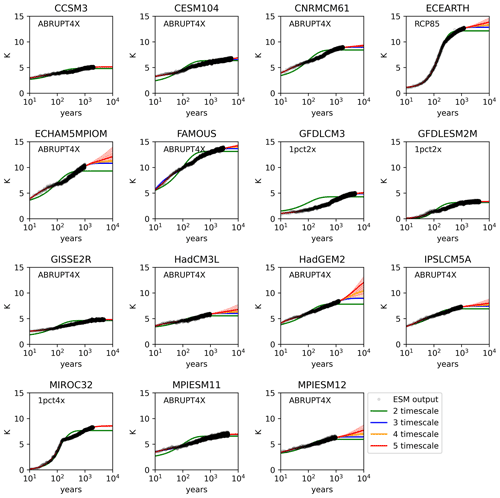Figure A1Results fitting multi-timescale models to output of LongRunMIP multi-thousand-year experiments for global mean surface temperature. Different colors represent different models as detailed in Table 1; shaded areas indicate the 5th–95th percentile range in the MCMC fit to the time series. Text indicates the model scenario used in the fit (as detailed in Table 3).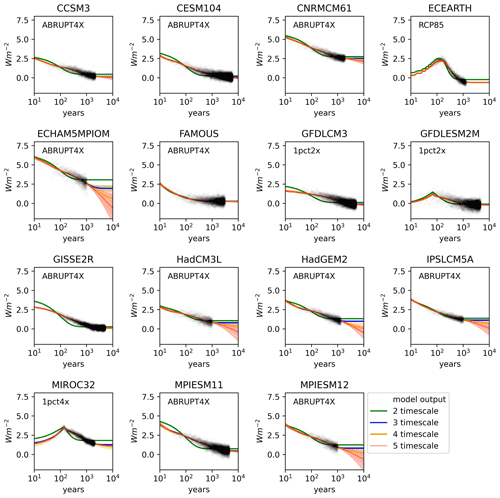Figure A2Results fitting multi-timescale models to output of LongRunMIP multi-thousand-year experiments for global top-of-atmosphere radiative imbalance. Different colors represent different models as detailed in Table 1; shaded areas indicate the 5th–95th percentile range in the MCMC fit to the time series. Text indicates the model scenario used in the fit (as detailed in Table 3).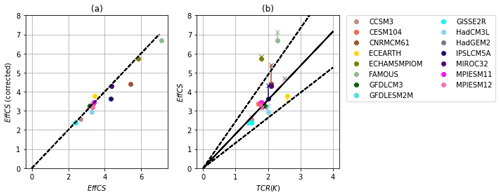Figure A3(a) Corrected EffCS plotted as a function of uncorrected EffCS. (b) EffCS (crosses) and corrected EffCS (circles) plotted as a function of transient climate response.

Code and data availability

All code to reproduce this study is available at https://doi.org/10.5281/zenodo.7181801 (). CMIP5 and CMIP6 source data are freely available and were here accessed on the Google Public Cloud https://console.cloud.google.com/storage/browser/cmip6 (last access: 1 November 2022). LongRunMIP data are available on request from Maria Rugenstein (maria.rugenstein@mpimet.mpg.de) or Jonah Bloch-Johnson (j.bloch-johnson@reading.ac.uk).

Author contributions

BMS performed all calculations, produced plots and wrote the main text. MR produced ESM data and co-wrote the main text.

Competing interests

The contact author has declared that neither of the authors has any competing interests.

Disclaimer

Publisher’s note: Copernicus Publications remains neutral with regard to jurisdictional claims in published maps and institutional affiliations.

Acknowledgements

We thank the modeling centers and scientists responsible for performing the experiments which made this study possible.

Financial support

This research has been supported by the H2020 European Research Council (grant nos. 101003536, 821003 and 101003687).

Review statement

This paper was edited by Christian Franzke and reviewed by Robbin Bastiaansen and one anonymous referee.

References

Andrews, T., Gregory, J. M., Webb, M. J., and Taylor, K. E.: Forcing, feedbacks and climate sensitivity in CMIP5 coupled atmosphere-ocean climate models, Geophys. Res. Lett., 39, L09712, https://doi.org/10.1029/2012GL051607, 2012. a

Andrews, T., Gregory, J. M., and Webb, M. J.: The dependence of radiative forcing and feedback on evolving patterns of surface temperature change in climate models, J. Climate, 28, 1630–1648, 2015. a

Andrews, T., Gregory, J. M., Paynter, D., Silvers, L. G., Zhou, C., Mauritsen, T., Webb, M. J., Armour, K. C., Forster, P. M., and Titchner, H.: Accounting for Changing Temperature Patterns Increases Historical Estimates of Climate Sensitivity, Geophys. Res. Lett., 45, 8490–8499, https://doi.org/10.1029/2018GL078887, 2018. a

Armour, K. C., Bitz, C. M., and Roe, G. H.: Time-Varying Climate Sensitivity from Regional Feedbacks, J. Climate, 26, 4518–4534, https://doi.org/10.1175/JCLI-D-12-00544.1, 2013. a

Bastiaansen, R., Dijkstra, H. A., and Heydt, A. S. v. d.: Projections of the Transient State-Dependency of Climate Feedbacks, Geophys. Res. Lett., 48, e2021GL094670, https://doi.org/10.1029/2021GL094670, 2021. a

Bloch-Johnson, J., Rugenstein, M., Stolpe, M. B., Rohrschneider, T., Zheng, Y., and Gregory, J. M.: Climate sensitivity increases under higher CO2 levels due to feedback temperature dependence, Geophys. Res. Lett., 48, e2020GL089074, https://doi.org/10.1029/2020GL089074, 2021. a, b

Caldeira, K. and Myhrvold, N. P.: Projections of the pace of warming following an abrupt increase in atmospheric carbon dioxide concentration, Environ. Res. Lett., 8, 034039, https://doi.org/10.1088/1748-9326/8/3/034039, 2013. a, b

Charney, J. G., Arakawa, A., Baker, D. J., Bolin, B., Dickinson, R. E., Goody, R. M., Leith, C. E., Stommel, H. M., and Wunsch, C. I.: Carbon dioxide and climate: a scientific assessment, National Academy of Sciences, Washington, DC, https://doi.org/10.17226/12181, 1979. a

Dunne, J. P., Winton, M., Bacmeister, J., Danabasoglu, G., Gettelman, A., Golaz, J.-C., Hannay, C., Schmidt, G. A., Krasting, J. P., Leung, L. R., Nazarenko, L., Sentman, L. T., Stouffer, R. J., and Wolfe, J. D.: Comparison of equilibrium climate sensitivity estimates from slab ocean, 150-year, and longer simulations, Geophys. Res. Lett., 47, e2020GL088852, https://doi.org/10.1029/2020GL088852, 2020. a

Eyring, V., Bony, S., Meehl, G. A., Senior, C. A., Stevens, B., Stouffer, R. J., and Taylor, K. E.: Overview of the Coupled Model Intercomparison Project Phase 6 (CMIP6) experimental design and organization, Geosci. Model Dev., 9, 1937–1958, https://doi.org/10.5194/gmd-9-1937-2016, 2016. a, b

Foreman-Mackey, D., Hogg, D. W., Lang, D., and Goodman, J.: emcee: The MCMC Hammer, Publ. Astron. Soc. Pac., 125, 306–312, https://doi.org/10.1086/670067, 2013. a

Forster, P., Storelvmo, T., Armour, K., Collins, W., Dufresne, J. L., Frame, D., Lunt, D. J., Mauritsen, T., Palmer, M. D., Watanabe, M., Wild, M., and Zhang, H.: The Earth’s Energy Budget, Climate Feedbacks, and Climate Sensitivity, in: Climate Change 2021: The Physical Science Basis. Contribution of Working Group I to the Sixth Assessment Report of the Intergovernmental Panel on Climate Change, edited by Masson-Delmotte, V., Zhai, P., Pirani, A., Connors, S. L., Péan, C., Berger, S., Caud, N., Chen, Y., Goldfarb, L., Gomis, M. I., Huang, M., Leitzell, K., Lonnoy, E., Matthews, J. B. R., Maycock, T. K., Waterfield, T., Yelekçi, O., Yu, R., and Zhou, B., book section 7, Cambridge University Press, Cambridge, United Kingdom and New York, NY, USA, https://www.ipcc.ch/report/ar6/wg1/downloads/report/IPCC_AR6_WGI_Chapter_07.pdf (last access: 14 December 2022), 2021. a

Forster, P. M.: Inference of climate sensitivity from analysis of Earth's energy budget, Annu. Rev. Earth Pl. Sc., 44, 85–106, 2016. a

Frame, D. J., Stone, D. A., Stott, P. A., and Allen, M. R.: Alternatives to stabilization scenarios, Geophys. Res. Lett., 33, L14707, https://doi.org/10.1029/2006GL025801, 2006. a

Geoffroy, O., Saint-Martin, D., Bellon, G., Voldoire, A., Olivié, D. J. L., and Tytéca, S.: Transient Climate Response in a Two-Layer Energy-Balance Model. Part II: Representation of the Efficacy of Deep-Ocean Heat Uptake and Validation for CMIP5 AOGCMs, J. Climate, 26, 1859–1876, https://doi.org/10.1175/JCLI-D-12-00196.1, 2013a. a

Geoffroy, O., Saint-Martin, D., Olivié, D. J. L., Voldoire, A., Bellon, G., and Tytéca, S.: Transient Climate Response in a Two-Layer Energy-Balance Model. Part I: Analytical Solution and Parameter Calibration Using CMIP5 AOGCM Experiments, J. Climate, 26, 1841–1857, https://doi.org/10.1175/JCLI-D-12-00195.1, 2013b. a

Gregory, J. M., Ingram, W. J., Palmer, M. A., Jones, G. S., Stott, P. A., Thorpe, R. B., Lowe, J. A., Johns, T. C., and Williams, K. D.: A new method for diagnosing radiative forcing and climate sensitivity, Geophys. Res. Lett., 31, L03205, https://doi.org/10.1029/2003GL018747, 2004. a, b

Gregory, J. M., Andrews, T., and Good, P.: The inconstancy of the transient climate response parameter under increasing CO2, Philos. T. R. Soc. A, 373, 20140417, https://doi.org/10.1098/rsta.2014.0417, 2015. a

Hansen, J., Lacis, A., Rind, D., Russell, G., Stone, P., Fung, I., Ruedy, R. and Lerner, J.: Climate Sensitivity: Analysis of Feedback Mechanisms, in: Climate Processes and Climate Sensitivity, edited by: Hansen, J. E. and Takahashi, T., https://doi.org/10.1029/GM029p0130, 1984. a

Hausfather, Z., Marvel, K., Schmidt, G. A., Nielsen-Gammon, J. W., and Zelinka, M.: Climate simulations: Recognize the “hot model” problem, Nature, 605, 26–29, https://doi.org/10.1038/d41586-022-01192-2, 2022. a, b

Hobbs, W., Palmer, M. D., and Monselesan, D.: An energy conservation analysis of ocean drift in the CMIP5 global coupled models, J. Climate, 29, 1639–1653, 2016. a, b, c, d

Huang, Y. and Bani Shahabadi, M.: Why logarithmic? A note on the dependence of radiative forcing on gas concentration, J. Geophys. Res.-Atmos., 119, 13683–13689, https://doi.org/10.1002/2014JD022466, 2014. a

IPCC: Climate Change 2013: The Physical Science Basis. Contribution of Working Group I to the Fifth Assessment Report of the Intergovernmental Panel on Climate Change, Cambridge University Press, Cambridge, United Kingdom and New York, NY, USA, https://doi.org/10.1017/CBO9781107415324, 2013. a

Irving, D., Hobbs, W., Church, J., and Zika, J.: A mass and energy conservation analysis of drift in the CMIP6 ensemble, J. Climate, 34, 3157–3170, 2021. a, b

Jarvis, A. and Li, S.: The contribution of timescales to the temperature response of climate models, Clim. Dynam., 36, 523–531, 2011. a

Jiménez-de-la Cuesta, D. and Mauritsen, T.: Emergent constraints on Earth’s transient and equilibrium response to doubled CO2 from post-1970s global warming, Nat. Geosci., 12, 902–905, 2019. a

Jonko, A. K., Shell, K. M., Sanderson, B. M., and Danabasoglu, G.: Climate feedbacks in CCSM3 under changing CO 2 forcing. Part I: Adapting the linear radiative kernel technique to feedback calculations for a broad range of forcings, J. Climate, 25, 5260–5272, 2012. a

Joos, F., Roth, R., Fuglestvedt, J. S., Peters, G. P., Enting, I. G., von Bloh, W., Brovkin, V., Burke, E. J., Eby, M., Edwards, N. R., Friedrich, T., Frölicher, T. L., Halloran, P. R., Holden, P. B., Jones, C., Kleinen, T., Mackenzie, F. T., Matsumoto, K., Meinshausen, M., Plattner, G.-K., Reisinger, A., Segschneider, J., Shaffer, G., Steinacher, M., Strassmann, K., Tanaka, K., Timmermann, A., and Weaver, A. J.: Carbon dioxide and climate impulse response functions for the computation of greenhouse gas metrics: a multi-model analysis, Atmos. Chem. Phys., 13, 2793–2825, https://doi.org/10.5194/acp-13-2793-2013, 2013. a

Knutti, R., Rugenstein, M. A. A., and Hegerl, G. C.: Beyond equilibrium climate sensitivity, Nat. Geosci., 10, 727–736, https://doi.org/10.1038/ngeo3017, 2017. a, b

Kuhlbrodt, T., Jones, C. G., Sellar, A., Storkey, D., Blockley, E., Stringer, M., Hill, R., Graham, T., Ridley, J., Blaker, A., Calvert, D., Copsey, D., Ellis, R., Hewitt, H., Hyder, P., Ineson, S., Mulcahy, J., Siahaan, A., and Walton, J.: The low-resolution version of HadGEM3 GC3. 1: Development and evaluation for global climate, J. Adv. Model. Earth Sy., 10, 2865–2888, 2018. a

Masson-Delmotte, V., Zhai, P., Pirani, A., Connors, S., Péan, C., Berger, S., Caud, N., Chen, Y., Goldfarb, L., Gomis, M., Huang, M., Leitzell, K., Lonnoy, E., Matthews, J., Maycock, T., Waterfield, T., Yelekçi, O., Yu, R., and Zhou, B.: Climate Change 2021: The Physical Science Basis. Contribution of Working Group I to the Sixth Assessment Report of the Intergovernmental Panel on Climate Change, Cambridge University Press, Cambridge, United Kingdom and New York, NY, USA, https://www.ipcc.ch/assessment-report/ar6/ (last access: 14 December 2022), 2021. a

Meehl, G. A., Senior, C. A., Eyring, V., Flato, G., Lamarque, J.-F., Stouffer, R. J., Taylor, K. E., and Schlund, M.: Context for interpreting equilibrium climate sensitivity and transient climate response from the CMIP6 Earth system models, Sci. Adv., 6, eaba1981, https://doi.org/10.1126/sciadv.aba1981, 2020. a, b

Meinshausen, M., Smith, S. J., Calvin, K., Daniel, J. S., Kainuma, M. L. T., Lamarque, J.-F., Matsumoto, K., Montzka, S. A., Raper, S. C. B., Riahi, K., Thomson, A., Velders, G. J. M., and van Vuuren, D. P. P.: The RCP greenhouse gas concentrations and their extensions from 1765 to 2300, Clim. Change, 109, 213–241, https://doi.org/10.1007/s10584-011-0156-z, 2011. a, b

Murphy, J.: Transient response of the Hadley centre coupled ocean-atmosphere model to increasing carbon dioxide. Part 1: control climate and flux adjustment, J. Climate, 8, 36–56, 1995. a

Myhre, G., Highwood, E. J., Shine, K. P., and Stordal, F.: New estimates of radiative forcing due to well mixed greenhouse gases, Geophys. Res. Lett., 25, 2715–2718, 1998. a, b

Nijsse, F. J. M. M., Cox, P. M., and Williamson, M. S.: Emergent constraints on transient climate response (TCR) and equilibrium climate sensitivity (ECS) from historical warming in CMIP5 and CMIP6 models, Earth Syst. Dynam., 11, 737–750, https://doi.org/10.5194/esd-11-737-2020, 2020. a

O'Neill, B. C., Tebaldi, C., van Vuuren, D. P., Eyring, V., Friedlingstein, P., Hurtt, G., Knutti, R., Kriegler, E., Lamarque, J.-F., Lowe, J., Meehl, G. A., Moss, R., Riahi, K., and Sanderson, B. M.: The Scenario Model Intercomparison Project (ScenarioMIP) for CMIP6, Geosci. Model Dev., 9, 3461–3482, https://doi.org/10.5194/gmd-9-3461-2016, 2016. a

Pfister, P. L. and Stocker, T. F.: State-Dependence of the Climate Sensitivity in Earth System Models of Intermediate Complexity, Geophys. Res. Lett., 44, 10–643, 2017. a

Pincus, R., Forster, P. M., and Stevens, B.: The Radiative Forcing Model Intercomparison Project (RFMIP): experimental protocol for CMIP6, Geosci. Model Dev., 9, 3447–3460, https://doi.org/10.5194/gmd-9-3447-2016, 2016. a

Proistosescu, C. and Huybers, P. J.: Slow climate mode reconciles historical and model-based estimates of climate sensitivity, Sci. Adv., 3, e1602821, https://doi.org/10.1126/sciadv.1602821, 2017. a, b, c, d

Rugenstein, M., Bloch-Johnson, J., Abe-Ouchi, A., Andrews, T., Beyerle, U., Cao, L., Chadha, T., Danabasoglu, G., Dufresne, J., Duan, L., Foujols, M., Frölicher, T., Geoffroy, O., Gregory, J., Knutti, R., Li, C., Marzocchi, A., Mauritsen, T., Menary, M., Moyer, E., Nazarenko, L., Paynter, D., Saint-Martin, D., Schmidt, G. A., Yamamoto, A., and Yang, S.: LongRunMIP: motivation and design for a large collection of millennial-length AOGCM simulations, B. Am. Meteorol. Soc., 100, 2551–2570, 2019. a, b, c, d

Rugenstein, M., Bloch-Johnson, J., Gregory, J., Andrews, T., Mauritsen, T., Li, C., Frölicher, T.L. , Paynter, D., Danabasoglu, G., Yang, S., Dufresne, J-L, Cao, L., Schmidt, G. A., Abe-Ouchi, A., Geoffroy, O. and Knutti, R.: Equilibrium climate sensitivity estimated by equilibrating climate models, Geophys. Res. Lett., 47, e2019GL083898, https://doi.org/10.1029/2019GL083898, 2020. a, b, c, d, e, f, g, h, i

Rugenstein, M. A. and Armour, K. C.: Three flavors of radiative feedbacks and their implications for estimating Equilibrium Climate Sensitivity, Geophys. Res. Lett., 48, e2021GL092983, https://doi.org/10.1029/2021GL092983, 2021. a, b, c, d

Rugenstein, M. A. A., Caldeira, K., and Knutti, R.: Dependence of global radiative feedbacks on evolving patterns of surface heat fluxes, Geophys. Res. Lett., 43, 9877–9885, https://doi.org/10.1002/2016GL070907, 2016. a

Sanderson, B.: Relating climate sensitivity indices to projection uncertainty, Earth Syst. Dynam., 11, 721–735, https://doi.org/10.5194/esd-11-721-2020, 2020. a, b, c

Sanderson, B.: benmsanderson/energybalance: Revised paper in ESD (0.2), Zenodo [code], https://doi.org/10.5281/zenodo.7181801, 2022. a

Senior, C. A. and Mitchell, J. F. B.: The time-dependence of climate sensitivity, Geophys. Res. Lett., 27, 2685–2688, https://doi.org/10.1029/2000GL011373, 2000. a

Sherwood, S., Webb, M. J., Annan, J. D., Armour, K. C., Forster, P. M., Hargreaves, J. C., Hegerl, G., Klein, S. A., Marvel, K. D., Rohling, E. J., Watanabe, M., Andrews, T., Braconnot, P., Bretherton, C. S., Foster, G. L., Hausfather, Z., von der Heydt, A. S., Knutti, R., Mauritsen, T., Norris, J. R., Proistosescu, C., Rugenstein, M., Schmidt, G. A., Tokarska, K. B., and Zelinka, M. D.: An assessment of Earth's climate sensitivity using multiple lines of evidence, Rev. Geophys., 58, e2019RG000678, https://doi.org/10.1029/2019rg000678, 2020.  a

Shields, C. A., Bailey, D. A., Danabasoglu, G., Jochum, M., Kiehl, J. T., Levis, S., and Park, S.: The low-resolution CCSM4, J. Climate, 25, 3993–4014, 2012. a

Smith, C. J., Forster, P. M., Allen, M., Leach, N., Millar, R. J., Passerello, G. A., and Regayre, L. A.: FAIR v1.3: a simple emissions-based impulse response and carbon cycle model, Geosci. Model Dev., 11, 2273–2297, https://doi.org/10.5194/gmd-11-2273-2018, 2018. a, b

Solomon, S., Daniel, J. S., Sanford, T. J., Murphy, D. M., Plattner, G.-K., Knutti, R., and Friedlingstein, P.: Persistence of climate changes due to a range of greenhouse gases, P. Natl. Acad. Sci. USA, 107, 18354–18359, 2010. a

Taylor, K. E., Stouffer, R. J., and Meehl, G. A.: An overview of CMIP5 and the experiment design, B. Am. Meteorol. Soc., 93, 485–498, 2012. a

Tokarska, K. B. and Gillett, N. P.: Cumulative carbon emissions budgets consistent with 1.5 C global warming, Nat. Clim. Change, 8, 296–299, https://doi.org/10.1038/s41558-018-0118-9, 2018. a

Tokarska, K. B., Stolpe, M. B., Sippel, S., Fischer, E. M., Smith, C. J., Lehner, F., and Knutti, R.: Past warming trend constrains future warming in CMIP6 models, Sci. Adv., 6, eaaz9549, https://doi.org/10.1126/sciadv.aaz9549, 2020. a

Wetherald, R. T., Stouffer, R. J., and Dixon, K. W.: Committed warming and its implications for climate change, Geophys. Res. Lett., 28, 1535–1538, 2001. a

Winton, M., Takahashi, K., and Held, I. M.: Importance of ocean heat uptake efficacy to transient climate change, J. Climate, 23, 2333–2344, 2010. a

Xia, J. Y., Luo, Y. Q., Wang, Y.-P., Weng, E. S., and Hararuk, O.: A semi-analytical solution to accelerate spin-up of a coupled carbon and nitrogen land model to steady state, Geosci. Model Dev., 5, 1259–1271, https://doi.org/10.5194/gmd-5-1259-2012, 2012. a

Zelinka, M. D., Myers, T. A., McCoy, D. T., Po-Chedley, S., Caldwell, P. M., Ceppi, P., Klein, S. A., and Taylor, K. E.: Causes of higher climate sensitivity in CMIP6 models, Geophys. Res. Lett., 47, e2019GL085782, https://doi.org/10.1029/2019GL085782, 2020. a

Zhang, M. and Huang, Y.: Radiative forcing of quadrupling CO2, J. Climate, 27, 2496–2508, 2014. a

Using ${R}_{\mathrm{extrap}}^{\mathrm{4}x}=-\mathrm{0.16}$ W m−2 rather than ${R}_{\mathrm{0}}^{\mathrm{CTRL}}=-\mathrm{0.01}$ W m−2 would result in a value of $\mathrm{\Delta }{T}_{\mathrm{best}-\mathrm{est}}=\mathrm{7.01}$ K, broadly consistent with EffCS and ΔTextrap.